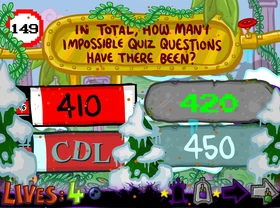## FANDOM

478 Pages

 The Impossible Quiz Book << Previous Question Question 149 Next Question >>Question 149Time Period

All eras represented in this Chapter, except Ancient Egypt.

Difficulty

Somewhat easy

Reference(s)

The entire Impossible Quiz series

Question 149 of The Impossible Quiz Book is the forty-ninth and penultimate question of Chapter 3, as well as the Book overall. At this point of the game, time has gone so haywire that all the historical eras represented throughout the Chapter have already clashed, just like the different Impossible Quiz eras, though it's surprisingly peaceful in here, with all ages seemingly blending together in perfect harmony, though this peace won't last for too long.

The last in the Book to follow the series' typical "question with four options" formula, the question states as follows: "In total, how many Impossible Quiz questions have there been?". The options you are given here are "410", "420", "CDL" (450) and "450".

The majority of people that get to this question seem to make the common mistake of only counting the main Quizzes of the series (that is, from The Impossible Quiz in 2007 to the current Chapter in 2012). However, there is one more Quiz not everyone is familiar with, which also counts for the amount of questions asked here: The Impossible Quiz Demo, released in 2004, which is the demo version of the first fully-fledged Quiz that pretty much everyone and their grannies have played.

The Demo had a total of 30 questions. These add to the 110 questions of the full version of the Quiz, the 120 of its sequel and the 150 of the Impossible Quiz Book, making together a total of 410 questions. This means that the answer to this question is the top left one, "410". Even if you didn't count the 30 questions of the Demo, this is the lowest possible option, so there is not much else to think about.

Note that the 10 additional questions (out of 40 total) from The Impossible Quiz Beta do not count, as this version was never released. The Impossible Quack has no questions because it was an April Fools joke. The 25 questions from The Impossible Quizmas also do not count, as the game had not yet been released.

## Trivia

• This question contains a visual trait of each of the different historical eras represented in this Chapter (with the exception of the Egyptian-themed era). Said traits are the following.
• The Prehistorical era: the two option boxes on the right, as well as the vines all over the screen.
• The Ice age: the trees in the foreground.
• The Industrial Revolution: the plate where the question's written.
• Germany of World War II: the two option boxes on the left
• The Roman era: the columns in the background and the text of the bottom-left option.
• Also represented here are the Monochrome and Retro eras of Chapter 2, seen in the font of the text in the second option and in the format of the question number, respectively.
• If the unreleased extended edition of the Demo, The Impossible Quiz Beta, had actually been released, the correct option would either be "420" (counting the 10 additional questions only, as the first 30 are exactly the same as in Demo) or "CDL"/"450" (counting all 40 questions, including the original 30).
• Both of the chapter's power-up locations can be seen in this question; The Fusestopper from Question 123 hiding in the bottom left (obscured by a tree), and the valve from Question 139 holding the Skip in the top right (can also be seen in Question 143). When clicked, the Fusestopper simply disappears, while the valve does nothing.
• This is one of several questions inquiring about the number of questions in the series.
• TIQ Question 74 asked how many questions there were in the original Quiz only.
• TIQ2 Question 21 asked how many questions there were in the "very first Impossible Quiz" (the Demo).
• The red flares from the lava can be seen falling from the top of this question's screen.
• This is the final skippable Question of the game.
• Assuming the player has been playing through the Quizzes in order, from the player's point of view there have technically only been 409 questions so far, as he or she will not have reached the final one yet.
Community content is available under CC-BY-SA unless otherwise noted.